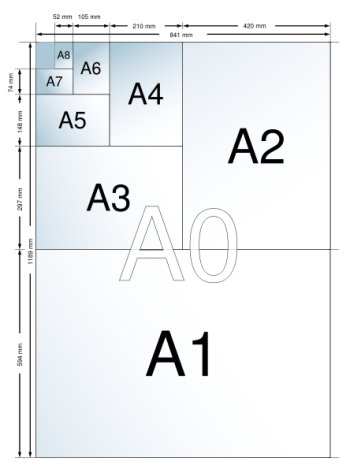# A4 Paper

I mentioned the A4 paper size (European standard) today in some of my classes and its special$\sqrt{2}$ ratio. It has the amazing property that if you cut it in half “hamburger” style it retains the ratio. Incredible, right? Beautiful, right? Nothing special about our 8.5″ x 11″ paper, that’s for sure. For extra bonus points, give a proof of this property (it’s two or three statements–short and simple).Elegantly, A0 paper is proportioned so that it has exactly 1 square meter of area. A1 is half that size, A2 half again, and so on. For more info about A4 paper, and diagrams, visit the Wikipedia page.

## 2 thoughts on “A4 Paper”

1.Drew Gallagher on said:

Proof (I admit Wikipedia helped a bit):

“sq2” means “square root of 2”.

Assume a rectangle of dimensions x and y, where x = y * sq2, and x and y are integers. The aspect ratio will be the quotient of longer and shorter side, x / y, which simplifies to (y * sq2) / y or sq2. Cut the rectangle in half so that y is now the longer side, and the shorter side measures x / 2. Now, the aspect ratio is y / (x / 2), so x / y = y / (x / 2). Simplify. x^2 / 2y = y. x^2 / 2 * y^2 = 1. x^2 / y^2 = 2. x / y = sq2. QED

2.Mr. Chase on said:

Nice! I knew I could count on you, Drew :-). Now you just need to learn how to tag with$LaTeX$ so it looks pretty, like this:$x=y\sqrt{2}$.